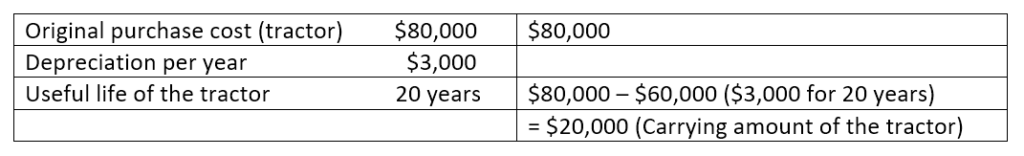# Carrying Amount

The value of an asset as reflected in a company’s book or balance sheet, minus its depreciation value

## What is the Carrying Amount?

The carrying amount is the value of an asset as reflected in a company’s book or balance sheet, minus the depreciation value of the asset. It is also called book value and is not necessarily the same as an asset’s fair value or market value.### Carrying Amount vs. Market Value

Carrying amount and market value differ in many ways, as listed below:

• Carrying amount is the value of an asset as it appears on the balance sheet and is acquired, after deducting its depreciation value and impairment expenses. The market value of an asset, on the other hand, depends on supply and demand, where if the demand is high, its value increases and if the demand is low, its value decreases.
• Carrying amount is based on the gradual depreciation of the value of a certain asset, which means that its value will change and decline through time. Market value is the value given to an asset when it is being sold in the open market.

### Example of Carrying Amount

Let’s say a company owns a tractor worth \$80,000 to be used for developing its newest land property. The said tractor’s depreciation value is \$3,000 per year and is expected to still be of use for 20 years. \$3,000 for 20 years is \$60,000. The carrying amount of the tractor is then \$20,000.### Example of Fair Value

Given the same tractor, its fair value will depend on the supply and demand in the market. If at the time it was sold in the market, the demand for tractors is high, it can be priced higher than its carrying value. The opposite can also be true if the demand goes down. The price of the tractor can go up or down, depending on how much buyers are willing to give for it.

### Carrying Value vs. Book Value

Many people use the terms carrying value and book value differently. But what they don’t know is that both terms are ultimately the same thing. The term carrying value refers to the value of the asset that is carried over to the end of its life, combined with its depreciation value. On the other hand, the term book value refers to the actual purchase cost of the asset that is recorded in the company’s book or balance sheet.

### How to Calculate for Carrying Amount

It is a very simple task to calculate for carrying amount, as shown in the example above. But to make it clearer, let’s explain it below:

• Take the original cost of purchasing the asset.
• Put together the depreciation cost for each year and multiply it with the number of years that the asset will be of use.
• Subtract the product from the original purchase price to get the carrying amount.

### Depreciation in the Carrying Amount

Depreciation is the lowering of the value of a tangible asset because of wear and tear. Tangible assets include buildings, equipment, furniture, and vehicles. One of the easiest and most commonly accepted methods of computing for depreciation is the straight-line depreciation method. Using the straight-line method, the same depreciation value is copied for every year, such as what was done in the above example wherein if the depreciation value for the first year is \$3,000, it would be the same value for the succeeding years.

The other method is the double-declining balance depreciation method, which is otherwise known as the 200% declining balance method. With the DDB method, the depreciation is faster than that of straight-line but will not make the depreciation value any bigger. It just means that depreciation is bigger in the early years but smaller in the later years.

CFI is the official provider of the global Financial Modeling & Valuation Analyst (FMVA)™ certification program, designed to help anyone become a world-class financial analyst. To keep advancing your career, the additional CFI resources below will be useful:

• Enterprise Value vs. Equity Value
• FCFF vs. FCFE
• Market Valuation Approach
• Valuation Methods